# Inductance

## (5) Growth and decay of current in L-R circuit

• Figure below shows a circuit containing resistance R and inductance L connected in series combination through a battery of constant emf E through a two way switch S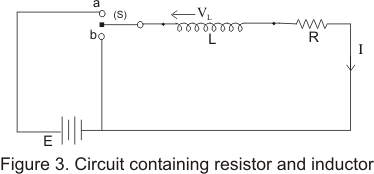• To distinguish the effects of R and L,we consider the inductor in the circuit as resistance less and resistance R as non-inductive
• Current in the circuit increases when the key is pressed and decreases when it is thrown to b

### (A) Growth of current

• Suppose in the beginning we close the switch in the up position as shown in below in the figure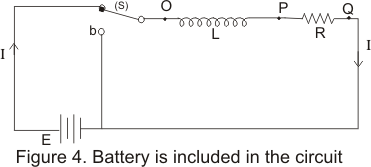• Switch is now closed and battery E,inductance L and resistance R are now connected in series
• Because of self induced emf current will not immediately reach its steady value but grows at a rate depending on inductance and resistance of the c circuit
• Let at any instant I be the c current in the circuit increasing from 0 to a maximum value at a rate of increase dI/dt
• Now the potential difference across the inductor is
Vop=LdI/dt
and across resistor is
Vpq=IR
Since
V=Vop+Vpq
so we have,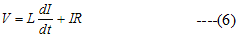Thus rate of increase of current would be,• In the beginning at t=0 when circuit is first closed current begins to grow at a rate,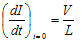from the above relation we conclude that greater would be the inductance of the inductor, more slowly the current starts to increase.
• When the current reaches its steady state value I ,the rate of increase of current becomes zero then from equation (7) we have,
0=(V-IR)/L
or,
I=V/R
From this we conclude that final steady state current in the circuit does not depend on self inductance rather it is same as it would be if only resistance is connected to the source
• Now we will obtain the relation of current as a function of time Again consider equation (6) and rearrange it so that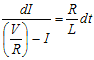let V/R=Imax ,the maximum current in the circuit .so we have• Integrating equation (8) on both sides we have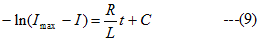where C is a constant and is evaluated by the value for current at t=0 which is i=0
so,
C=-ln(V/R)=-ln Imax
putting this value of C in equation (9) we get,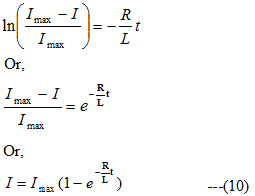This equation shows the exponential increase of current in the circuit with the passage of time

• Figure below shows the plot of current versus time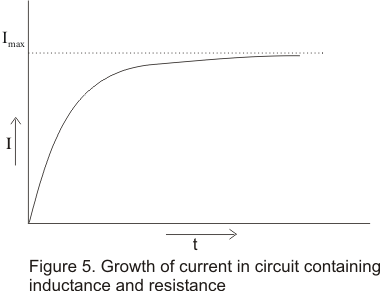• If we put t=τL=L/R is equation 10 then,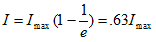Hence, the time in which the current in the circuit increases from zero to 63% of the maximum value of Imax is called the constant or the decay constant of the circuit.
• For LR circuit, decay constant is,
τL=L/R                                 ---(11)
• Again from equation (8),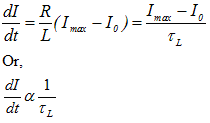This suggests that rate of change current per sec depends on time constant.
• Higher is the value of decay constant ,lower will be the rate of change of current and vice versa.

### (B) Decay of current

• When the switch S is thrown down to b as shown below in the figure ,the L-R circuit is again closed and battery is cut off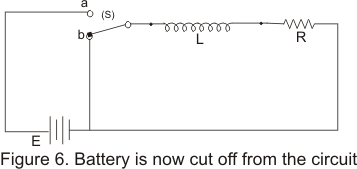• In this condition the current in the circuit begins to decay
• Again from equation (8) since V=0 this time, so the equation for decay is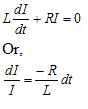Integrating on both sidesIn this case initially at time t=0 current I=Imax so
C1=ln I0
Putting this value of C1 in equation (12)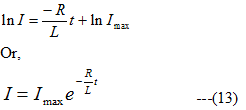Hence current decreases exponentially with time in the circuit in accordance with the above equation after the battery are cutoff from the circuit.
• Figure below shows the graph between current and time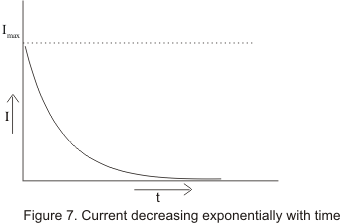• If in equation (13)
t=τL=L/R
then
I=Imaxe-1=.37Imax
hence the time in which the current decrease from the maximum value to 37% of the maximum value Imax is called the time constant of the circuit
• From equation (13) it is clear that when R is large ,current in the L-R circuit will decrease rapidly and there is a chance of production of spark
• To avoid this situation L is kept large enough to make L/R large so that current can decrease slowly
• For large time constant the decay is slow and for small time constant the decay is fast

Note to our visitors :-

Thanks for visiting our website.
DISCLOSURE: THIS PAGE MAY CONTAIN AFFILIATE LINKS, MEANING I GET A COMMISSION IF YOU DECIDE TO MAKE A PURCHASE THROUGH MY LINKS, AT NO COST TO YOU. PLEASE READ MY DISCLOSURE FOR MORE INFO.